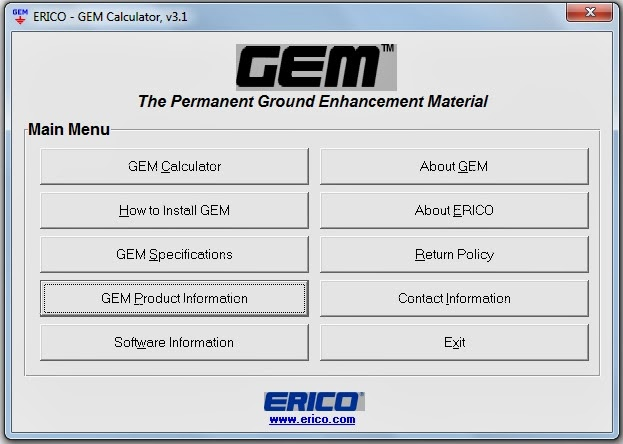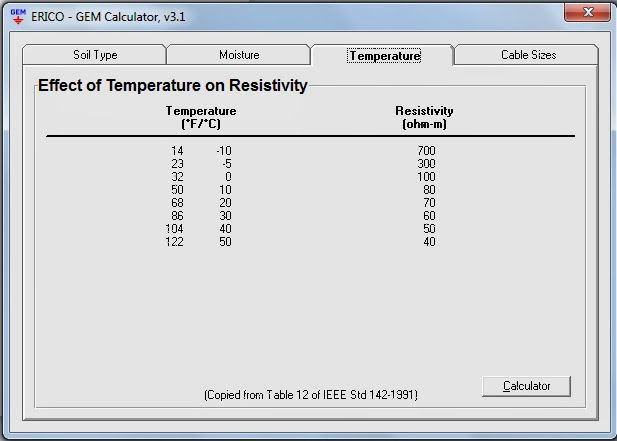### Grounding Design Calculations – Part Nine

I indicated that the Earthing Systems Design Steps process has (3) main steps:

 Earthing Systems Design Steps A grounding system design process has (3) main steps: Data Collection, Data Analysis, Grounding Design Calculations.

And I explained the first step: Data Collection in the following Articles:

I explained the second step: Data Analysis in the following Articles:

And I explained What we are going to design for grounding system in any building in the following Articles:

And, in Article
Grounding Design Calculations – Part One ", I indicated the following:

 Grounding System Design Calculations according to type of the building The procedures for performing the Grounding System Design Calculations can differ slightly according to the type of the building as follows: Domestic, commercial and industrial premises, High and medium voltage electricity substations.

 First: Domestic, commercial and industrial premises We mean by domestic, commercial and industrial premises, all installations up to 1,000 V ac and 1,500 V dc - between phases, with some minor exceptions.

And I started discussion for Methods of Grounding Design Calculations of Domestic, commercial and industrial premises as follows:

 Methods of Grounding Design Calculations There are many methods can be used for performing Grounding System Design Calculations But the common methods are: Equations Method, Nomographs Method, Excel Spreadsheets Method, Tables Method, Online Earthing Calculators Method, Software Programs Method.

Grounding Design Calculations – Part Two ", I explained the first method of grounding design calculations: Equations Method and solved examples.

And I explained the second method of grounding design calculations: Nomographs Method in Article
Grounding Design Calculations – Part Three".

Also, I explained the third method of grounding design calculations: Excel Spreadsheets Method in Article
Grounding Design Calculations – Part Four ".

And I explained the forth method of grounding design calculations: By using Tables In Articles
Grounding Design Calculations – Part Five "

Also, I explained the fifth method of grounding design calculations: By using Online Earthing Calculators in Articles
Grounding Design Calculations – Part Seven " and " Grounding Design Calculations – Part Eight ".

Today, I will explain the Sixth Method of Grounding Design Calculations: Software Programs Method.

 Sixth Method: Software Programs Method Actually, there are many software programs for Grounding Design Calculations as: Etap, SES Software Package, CDEGS Integrated Software for Power System Grounding/Earthing, Electromagnetic Fields and Electromagnetic Interference, CYMGRD, Substation Grounding Program, GEM from Erico, Etc… But Unfortunately, most of these programs are not for free, you can download a demo with free trial period or less functions/capabilities. The only free software program for Grounding Design Calculations is Erico-Gem and because of this, it will be explained in this article.

 Erico – GEM Calculator

 1- Benefits from using this program This program calculates resistance to Earth with or without the use of ERICO's Ground Enhancement Material (GEM). This program estimates the quantity of GEM required and is valid for horizontal and vertical Earth electrodes. Also incorporated within the program are installation instructions for both horizontal and vertical Earth electrodes using GEM.

2- Basis of Calculation

• The formulas used in this program are based on accepted formulas which are the basis of IEEE publications
• The formulas used for calculating resistance to Earth are based on modified formulas from Dwight for horizontal electrodes and from Sunde for vertical electrodes.
• The modified formula assumes the top of the vertical electrode is near the surface.
• When more than one vertical electrode are calculated, the calculations assume equally spaced ground rods in a straight line and that the spacing between the ground rods is equal to or greater than the length of the vertical Earth electrode.
• The formula for calculating the resistance to Earth for the horizontal Earth electrode assumes the electrode is in a straight line.
• Both of the horizontal and vertical resistance to Earth formulas are for DC or low frequency. Both sets of equations use, as an input, the diameter of the cable as well as that of the surrounding GEM material to calculate the resistance to Earth.
• The modified formulas assume uniform soil resistivity.
• The contact resistance between the conductor and the surrounding GEM material is ignored in calculating resistance to Earth.

###### Notes:

• The use of this program and the selection of the input parameters require an engineering judgement for each specific application. Calculations used within this program are based on approximations which may or may not apply to specific applications.
• The calculated amount of GEM is very conservative. The results are rounded to the nearest 25 pound bag of GEM. In some cases, you will need less GEM material than the program results indicate.

 3- Program Interfaces The programs include group of interfaces or windows as follows: Language interface, Main menu interface.

 3.1 Language interface In this interface, we need to Select the Language to proceed and The available languages are: English, Espanol, Francais, Deutsch.Erico-GEM Main Menu Interface

Main menu interface includes the following buttons:

1. GEM Calculator,
2. How to Install GEM,
3. GEM Specifications,
4. GEM Product Information,
5. Software Information,
8. Return Policy,
9. Contact Information,
10. Exit.

The only important button for us is "GEM Calculator" Button while other buttons introduce written information or instructions that need to be reviewed and understood.

GEM Calculator InterfaceErico-GEM Calculator Interface

The GEM Calculator includes the following buttons:

1. Single Ground Rod (without GEM),
2. Single Ground Rod (with GEM),
3. Multiple Ground Rods in a Line (without GEM),
4. Multiple Ground Rods in a Line (with GEM),
5. Bare Ground Wire in a Line (without GEM),
6. Bare Ground Wire in a Line (with GEM),
7. Bare Ground Wire in a Ring (without GEM),
8. Bare Ground Wire in a Ring (with GEM),

General Notes For All Buttons In GEM Calculator Interface

• Choose Metric or English units, prior to entering variables, by putting the dot beside required unit system. The English units are feet and inch while the Metric units are meter and cm.
• Regardless of the input units selected (English or Metric), the units for resistivity must be in ohm-m.
• Use the Reference button in below of the calculation window for getting the following information:

1- Resistivity (ohm-m) of soil based on soil type: copied from table 10 of IEEE std 142 -1991,Resistivity (ohm-m) of soil based on soil type

2-Effect of Moisture Content on Resistivity:
copied from table 11 of IEEE std 142 -1991,Effect of Moisture Content on Resistivity

3- Effect of temperature on Resistivity: copied from table 12 of IEEE std 142 -1991,Effect of temperature on Resistivity

4- Cable Diameter Equivalents: give the equivalent diameter in inches and cm for a given cable cross section area in Kcmil, AWG and mm2.Cable Diameter Equivalents

• For recalculating with new variables, press Clear Button then enter the new variables.
• For printing the calculation window, press Print button.

 Calculation#1: for Single Ground Rod (without GEM) Step#1: Enter the following data: Resistivity of soil in (ohm-m), Length of ground rod in (ft or m): must be at least (3) feet or (0.914) meter, Diameter of ground rod in (inch or cm). Step#2: Press the calculate button. Step#3: Results step: you will get the electrode resistance in (ohms).

 Calculation#2: for Single Ground Rod (with GEM) Step#1: Enter the following data: Resistivity of soil in (ohm-m), Length of ground rod in (ft or m): must be at least (4) feet or (1.22) meter, Diameter of ground rod in (inch or cm), Diameter of Hole in (inch or cm): must be between (4) inches/ (10) cm and (12) inches/ (30.5) cm. Step#2: Press the calculate button. Step#3: Results step: you will get the electrode resistance in (ohms) and the required number of GEM Bags.

 Calculation#3: for Multiple Ground Rods in a Line (without GEM) Step#1: Enter the following data: Resistivity of soil in (ohm-m), Length of ground rods in (ft or m): must be at least (3) feet or (0.914) meter, Diameter of ground rods in (inch or cm), Spacing Between Rods in (ft or m): rod spacing must be at least the length of the ground rod, Number of Rods: must be greater than one. Step#2: Press the calculate button. Step#3: Results step: you will get the electrode resistance in (ohms).

 Calculation#4: for Multiple Ground Rods in a Line (with GEM) Step#1: Enter the following data: Resistivity of soil in (ohm-m), Length of ground rods in (ft or m): must be at least (4) feet or (1.22) meter, Diameter of ground rods in (inch or cm), Diameter of Holes in (inch or cm): must be between (4) inches/ (10) cm and (12) inches/ (30.5) cm. Spacing Between Rods in (ft or m): rod spacing must be at least the length of the ground rod, Number of Rods: must be greater than one. Step#2: Press the calculate button. Step#3: Results step: you will get the electrode resistance in (ohms) and the required number of GEM Bags. Note: Wire does not contribute with the final electrode resistance value.

 Calculation#5: for Bare Ground Wire in a Line (without GEM) Step#1: Enter the following data: Resistivity of soil in (ohm-m), Length of Wire in (ft or m): must be at least (5) feet or (1.54) meter, Diameter of Wire in (inch or cm). Step#2: Press the calculate button. Step#3: Results step: you will get the electrode resistance in (ohms).

 Calculation#6: for Bare Ground Wire in a Line (with GEM) Step#1: Enter the following data: Resistivity of soil in (ohm-m), Length of Wire in (ft or m): must be at least (5) feet or (1.54) meter, Diameter of Wire in (inch or cm), Width of Trench in (inch or cm), Thickness of GEM in (inch or cm): must be at least (2) inch/ (5) cm and no more than (12) inch/ (30.5) cm. Step#2: Press the calculate button. Step#3: Results step: you will get the electrode resistance in (ohms) and the required number of GEM Bags.

 Calculation#7: Bare Ground Wire in a Ring (without GEM) Step#1: Enter the following data: Resistivity of soil in (ohm-m), Diameter of Wire in (inch or cm), Depth of Wire in (ft or m), Diameter of Ring in (ft or m): should be at least (10) feet or (3.04) meter. Step#2: Press the calculate button. Step#3: Results step: you will get the electrode resistance in (ohms).

 Calculation#8: Bare Ground Wire in a Ring (with GEM) Step#1: Enter the following data: Resistivity of soil in (ohm-m), Diameter of Wire in (inch or cm), Depth of Wire in (ft or m), Width of Trench in (inch or cm), Thickness of GEM in (inch or cm): must be at least (2) inch/ (5) cm and no more than (12) inch/ (30.5) cm. Diameter of Ring in (ft or m): should be at least (10) feet or (3.04) meter. Step#2: Press the calculate button. Step#3: Results step: you will get the electrode resistance in (ohms) and the required number of GEM Bags.GEM Materials

• Developed in 1992, GEM (Ground Enhancement Material) is a superior conductive material that solves toughest grounding problems. GEM improves grounding effectiveness regardless of soil conditions. It is the ideal material to use in areas of poor conductivity such as rocky ground, mountain tops, and sandy soil.
• GEM is also the answer in situations where ground rods can't be driven. Or where limited land area makes adequate grounding difficult with conventional methods.